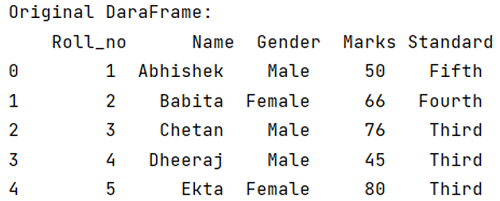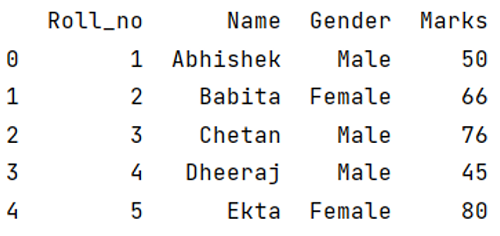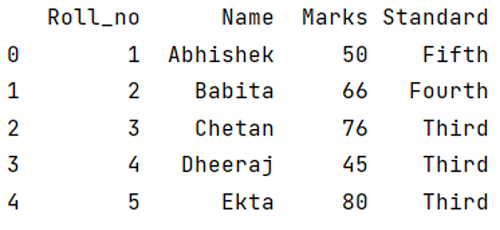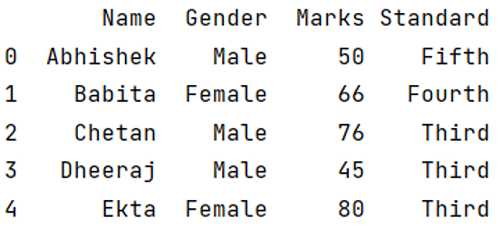# Selecting/excluding sets of columns in pandas

Learn how to select/exclude sets of columns in pandas?
Submitted by Pranit Sharma, on May 04, 2022

Columns are the different fields that contain their particular values when we create a DataFrame. We can perform certain operations on both rows & column values. Suppose we want to display all the columns except some specified columns.

## Problem statement

Given a DataFrame, we have to select/exclude sets of columns in pandas.

## Selecting/excluding sets of columns in pandas

For this purpose, we use DataFrame.loc[] property with the specific conditions and/or slicing. The DataFrame.loc[] property will read the sliced index, colon (:) means starting from the first column, DataFrame.columns will return all the columns of a DataFrame and define a specified column name after the conditional operator (!=) will return all the column names except the one which is specified inside the method.

### Syntax

```DataFrame.loc[:, df.columns!= 'column_name']
```

Here, the DataFrame.loc() property will read the sliced index, colon (:) means starting from the first column, DataFrame.columns will return all the columns of a DataFrame and define a specified column name after the conditional operator (!=) will return all the column names except the one which is specified inside the method.

Note

To work with pandas, we need to import pandas package first, below is the syntax:

```import pandas as pd
```

Let us understand with the help of an example:

## Creating a DataFrame in Python

```# Importing pandas package
import pandas as pd

# Creating a dictionary
d = {
'Roll_no': [ 1,2,3,4,5],
'Name': ['Abhishek', 'Babita','Chetan','Dheeraj','Ekta'],
'Gender': ['Male','Female','Male','Male','Female'],
'Marks': [50,66,76,45,80],
'Standard': ['Fifth','Fourth','Third','Third','Third']
}

# Creating a DataFrame
df = pd.DataFrame(d)

# Display original DataFrame
print("Original DataFrame:\n",df,"\n\n")
```

The output of the above program is:## Examples of selecting/excluding sets of columns in pandas

Now, we will print the DataFrame by excluding some columns

### Example 1

```# 1] Display columns according to our requirements

print(df.loc[:,df.columns!='Standard'])
```

The output of the above program is:### Example 2

```# 2] Display columns according to our requirements

print(df.loc[:,df.columns!='Gender'])
```

The output of the above program is:### Example 3

```# 3] Display columns according to our requirements

print(df.loc[:,df.columns!='Roll_no'])
```

The output of the above program is: